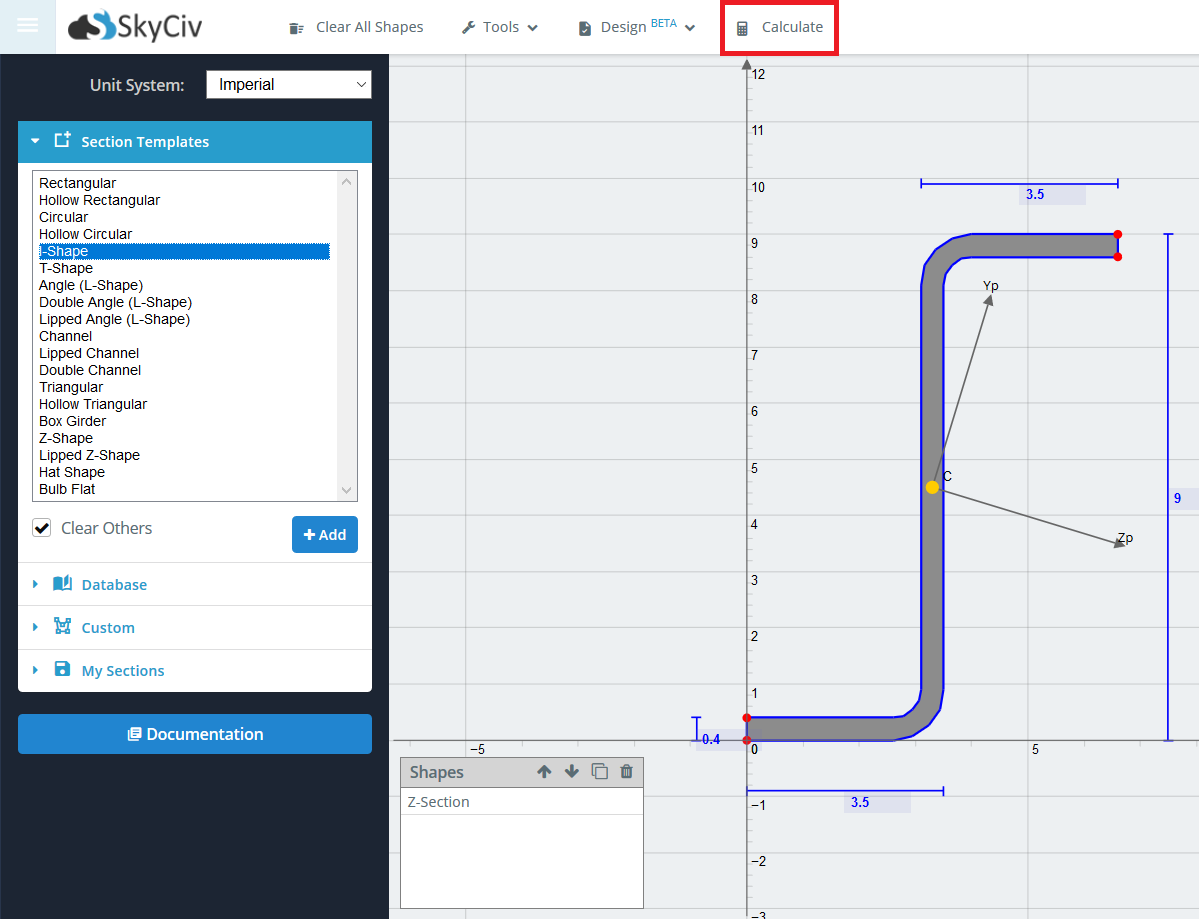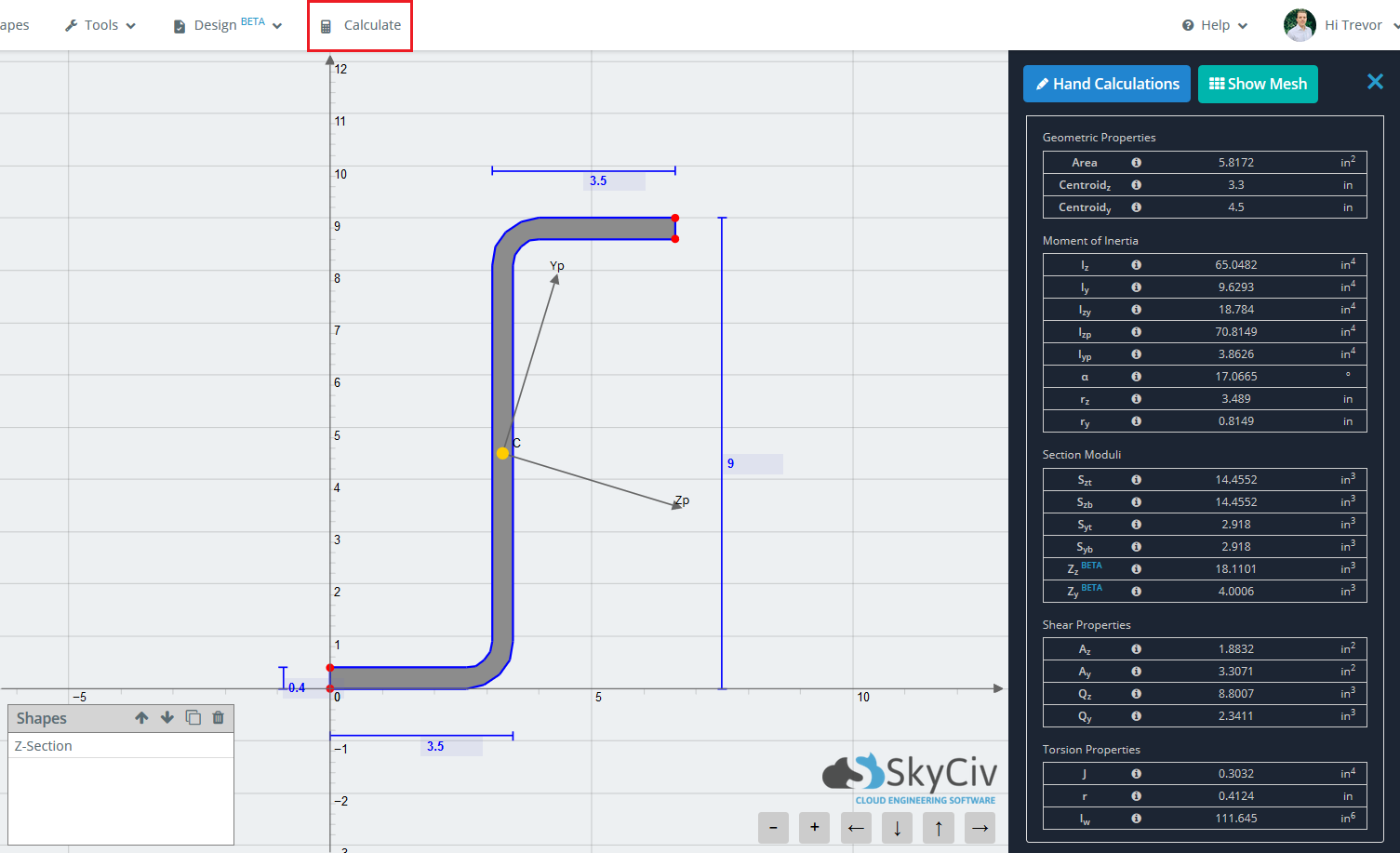Documentação SkyCiv

Seu guia para o software SkyCiv - tutoriais, guias de instruções e artigos técnicos

1. Casa
2. SkyCiv Section Builder

The main purpose of the Section Builder is to calculate the various section properties of your section. The standard results refer to the results of a section when ignoring material property differences.

When you’ve finished building your section, simply click ‘Calculateon the top toolbar to determine the section properties. Note that the short wait time associated with each calculate is due to the Finite Element Analysis and Meshing that occurs in the background in order to solve for torsion constant, raio de torção, e constante de empenamento. Usando um método FEA para calcular essas propriedades, uma maior precisão é alcançada. Less robust programs only use approximate equations on the standard sections for these properties and fail to solve when a non standard section (como a viga cruzada abaixo) é introduzido.Os resultados são geralmente divididos em 5 Categorias principais.

• Área
• Centroid (COM)
• Centroid (Y)
• Moment of Inertia Properties
• A partir de – Moment of Inertia about the Z-axis
• Iy – Moment of Inertia about the Y-axis
• Izy – Momento de inércia
• IzpMoment of Inertia about the principal Z-axis
• IypMoment of Inertia about the principal Y-axis
• uma – angle of rotation of principal axis
• Módulos de seção
• SztElastic Section Modulus about the Z-axis to the top of the section
• SzbElastic Section Modulus about the Z-axis to the bottom of the section
• SytElastic Section Modulus about the Y-axis to the top of the section
• SybElastic Section Modulus about the Y-axis to the bottom of the section
• ZzPlastic Section Modulus about the Z-axis
• ZyPlastic Section Modulus about the Y-axis
• AzShear Area about Z axis
• AyShear Area about Y axis
• Qz – Static Moment of Area about the Z neutral axis
• Qy – Static Moment of Area about the Y neutral axis
• Torsion Properties
• J – Constante de Torção
• r – Raio de torção
• Iw – Constante de empenamentoEste artigo foi útil para você?